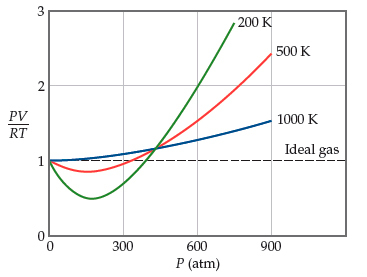# Problem: Explain the negative deviation from ideal gas behavior of N2 below 300 atm in the figure on the left.

🤓 Based on our data, we think this question is relevant for Professor Atwood's class at UB.

###### FREE Expert Solution

In this problem, we are asked to explain the negative deviation from the ideal gas behavior of N2 below 300 atm.

The deviation from the ideal gas behavior is expressed introducing a factor in the ideal gas equation.

This is the compressibility factor (Z) which is expressed as:

$\overline{){\mathbf{Z}}{\mathbf{=}}\frac{\mathbf{PV}}{\mathbf{nRT}}}$

Where:
For ideal gas:  Z = 1
For real gas: Z 1

In the case of real gases:###### Problem Details

Explain the negative deviation from ideal gas behavior of N2 below 300 atm in the figure on the left.